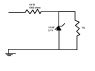# Circuit with a zener diode

dancergirlie

## Homework Statement

What would happen if the load resistor was short-circuited. What would happen if the load resister was removed?

-see attached diagram-

## Homework Equations

P=Iin^2*R
Pz=IzVz
=(Iin-Iout)Vz=[((Vin-Vz)/R)-Iout]Vz

## The Attempt at a Solution

I'm not sure about short circuited, but I think that if it is removed then the current Iout would be greater, which would give us a smaller power rating, but I'm not sure how to figure it out!

Any help would be great!

#### Attachments

•Untitled.png
2.1 KB · Views: 693

Mentor
Without a supply voltage being specified you can only answer in generalities. So why not put in a hypothetical value for the supply voltage (say 10V) and work out the details when you short or remove the load resistor. You can "translate" your results back to generalities for the response.

dancergirlie
sorry supply is 12v

Mentor
sorry supply is 12v

Take the case where RL is shorted. What's the voltage drop across the zener? How about the series resistor (1000 Ω resistor)?

dancergirlie
I have no idea... I would need to know the current through the zener, and I don't know how to figure that out :(

dancergirlie
oh wait, well i have the power as 1/4 W and the voltage as 5.1V, so wouldn't the current be .25/5.1V. But, that doesn't make sense though cause the V is given, so isn't that the voltage drop across the zener?

Mentor
If RL is shorted, so is the zener. What's the voltage across a short circuit?

dancergirlie
well if the zener is shorted then it would have no current running through it, so then the voltage across it would be zero

Mentor
well if the zener is shorted then it would have no current running through it, so then the voltage across it would be zero

Correct. So that leaves you with a very simple circuit comprising the voltage supply and the 1000Ω 1/4 watt resistor. What's the power being dissipated in the resistor?

dancergirlie
Well it would have to be all of the voltage drop through the resistor, so I'm guessing that it would also dissipate all the power. So would that be 1/4 watt or would we do V^2/R so it would be 144/1000 W?

Mentor
Definitely do the V2/R or I2R thing. The "1/4 watt" on the circuit diagram specifies the safe rating for the part (the maximum continuous power dissipation that the part is rated to handle).

dancergirlie
So, what if the resistor was 100 ohms? Then the power rating would be 144/100, which would be 1.44 watts. If we have a a 1/4 watt resistor, and a power greater than that being dissipated by it, what would happen to the resistor?

Mentor
So, what if the resistor was 100 ohms? Then the power rating would be 144/100, which would be 1.44 watts. If we have a a 1/4 watt resistor, and a power greater than that being dissipated by it, what would happen to the resistor?

The magic smoke would escape. Sizzle, pop! Evil odor.

Actually, the effect may not be quite so drastic depending upon how much it's rating is exceeded. If not by much, it may only run very hot. And it will probably change its intrinsic value over time (1000Ω becoming something smaller) as thermal stresses act on it.

dancergirlie
So, now if the load resistor is taken out, we would have a diode that would still have no current running through it (because the direction of the arrow in the zener). So wouldn't the power being dissipated by the resistor be the same?

Mentor
So, now if the load resistor is taken out, we would have a diode that would still have no current running through it (because the direction of the arrow in the zener). So wouldn't the power being dissipated by the resistor be the same?

Zeners are normally operated in the reverse bias region. They are actually designed to operate with the voltage exceeding the reverse breakdown voltage of the diode junction.

Until the breakdown voltage is exceeded the current though the part is essentially nil. After the breakdown voltage is exceeded, there is a failure of the diode junction to prevent current flow in the reverse bias mode. The number of free charge carriers available to carry current through the zener increases dramatically.

If you wish, you can think of the zener in terms of an equivalent circuit comprising a normal diode, a small series resistance, and a series voltage supply equal to the zener voltage. The diode only conducts when an external voltage across the three exceeds the voltage of the "internal" supply. Then it behaves like just a small resistor.

Now, back to the circuit. When the load resistor is removed, the zener will be handling all of the current. There's plenty of available voltage to "turn on" the zener, since it is connected to the voltage supply through the series resistor. The voltage drop across the zener will be, well, the zener voltage! So you should be able to calculate the drop across the series resistor and hence the current through the parts.